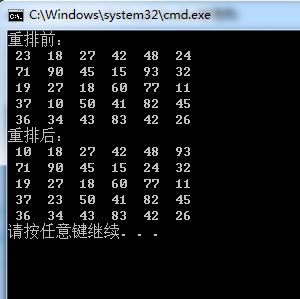###### qq_35021563

2016-05-17 00:20 阅读 2.1k

# 一道简单的C语言程序设计题

• 点赞
• 写回答
• 关注问题
• 收藏
• 复制链接分享

#### 3条回答默认 最新

• `````` #include <stdlib.h>
#include <stdio.h>
#include <time.h>

int main (){
int i, j, k, min_x = 0, min_y = 0, max_x = 0, max_y = 0, arr;
srand((unsigned)time(NULL));

//产生随机数
printf("重排前：\n");
for(i = 0; i < 5; i++)
{
for(j = 0; j < 6; j++)
{
arr[i][j] = rand() % 91 + 10;
printf("%3d ", arr[i][j]);
}
printf("\n");
}

for(i = 0; i < 5; i++)
{
for(j = 0; j < 6; j++)
{
if(arr[i][j] > arr[max_x][max_y])
{
max_x = i;
max_y = j;
}
else if(arr[i][j] < arr[min_x][min_y])
{
min_x = i;
min_y = j;
}
}
}

//最大值元素和右上角元素对调
k = arr[max_x][max_y];
arr[max_x][max_y] = arr;
arr = k;

//最小值元素和左上角元素对调
k = arr[min_x][min_y];
arr[min_x][min_y] = arr;
arr = k;

printf("重排后：\n");
for(i = 0; i < 5; i++)
{
for(j = 0; j < 6; j++)
printf("%3d ", arr[i][j]);
printf("\n");
}
}

``````点赞 2 评论 复制链接分享
• 这机器人小D ，也是无奈了，，，， 楼主还是先学C语言吧，这题C的一些基本操作都可以解决

点赞 评论 复制链接分享
• 我觉得思路可以是这样的：首先对每一行
====>将最大值放到左端，将最小值放到右端；
====>对第一列和最后一列进行值比较，将最大的值放大上面，将最小的值放到下面。

点赞 评论 复制链接分享